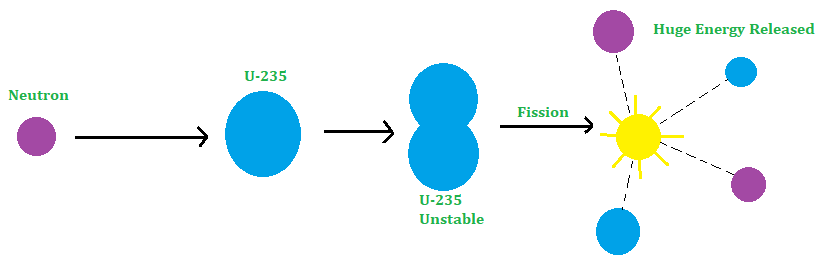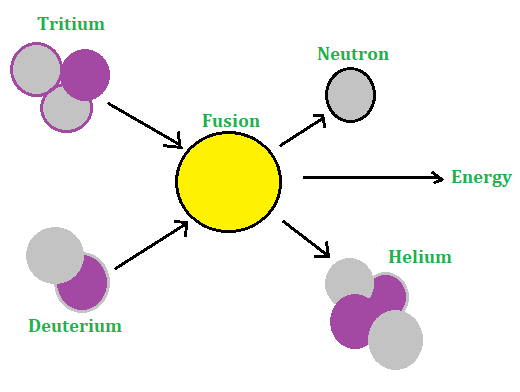GFG App
Open AppBrowser
Continue

The nucleus of an atom is very small. But it is a matter of fact that a single tiny nucleus of an atom will produce a massive amount of nuclear energy? What is the source of this electricity, and how does this is obtained? Let’s take a closer look at nuclear technology and how it differs from other types of energy.

### What is Nuclear Energy?

Nuclear energy is the energy stored within the nucleus of an atom, which is a small particle that makes up all matter in the universe. The mass of an atom is normally concentrated in the nucleus centre. The nucleus is composed of two subatomic particles: neutrons and protons. Bonds that hold atoms together provide a huge amount of energy.

Antonie Henri Becquerel, a French physicist discovered that Uranium emits radiation spontaneously. The spontaneous emission of radiation by uranium or heat elements is known as radioactivity. A substance or element that shows the property of radioactivity is known as a radioactive substance or radioactive element.

In 1897, Rutherford found that the radiation emitted by radioactive elements are of three types:

(i) Alpha particle: The alpha particle is a helium nucleus i.e. a helium atom that has lost its two orbital electrons.

(ii) Beta particle: The Beta particles are the streams of fast-moving electrons.

(iii) Gamma rays: The gamma rays are simply the radiation of small wavelengths.

When a radioactive substance emits radiation, its mass decreases. Suppose M be the mass of a radioactive substance before emitting the radiation and M be the mass of the substance after emitting the radiation. Therefore, the decrease in the mass of the substance is given by:

△m = (M – M’)

This decrease in mass (△m) is converted into energy E according to Einstein’s mass-energy relation,

E = △mc2

where c the speed of light in a vacuum which is equal to 3 × 108 m/s and the energy E is known as nuclear energy.

Unit of Nuclear Energy: Nuclear energy is expressed in Electron-volt (eV)

Therefore, 1 eV is defined as the energy acquired by an election while passing through a potential difference of one volt. The nuclear energy of 1 eV is equivalent to Joules:

1 eV = 1.6 x 10-19 C × 1 V = 1.6 × 10-19 CV or 1.6 × 10-19 J.

Another unit for nuclear energy is Mega electron-volt (MeV). The nuclear energy of 1 MeV is defined as:

1 MeV = 106 eV = 106 × 1.6 × 10-19 J = 1.6 × 10-13 J.

### Nuclear Reactions

Atomic nuclei undergo modifications as a result of nuclear reactions, which result in changes in the atom itself. Nuclear reactions change one aspect into something entirely new. However, nuclear scattering happens as a nucleus interacts with other particles and then splits without changing the properties of the other nuclei.

There are two kinds of nuclear reactions that can produce nuclear energy:

(i) Nuclear Fission: Nuclear fission is the mechanism by which a radioactive atom’s heavy nucleus, such as plutonium or uranium, breaks into smaller nuclei when bombarded by low-energy neutrons.

(ii) Nuclear Fusion: The reaction which requires the fusion of two light elements to produce a heavier element and the release of energy is called the nuclear fusion.

## Nuclear Fission

Otto Hahn and Strassman found that Uranium-235 bombarded by a neutron splits up into two comparatively lighter nuclei. This process is known as nuclear fission. The neutrons used in the fission of the Uranium nucleus have low energy and are known as thermal neutrons. During the fission of uranium, two or three neutrons are also emitted along with the release of a large amount of energy besides the products of the fission.

Thus, the process of splitting a heavy nucleus into two comparatively lighter nuclei along with the release of a large amount of energy when bombarded with a thermal neutron is called nuclear fission

Nuclear fission of 92U235 when bombarded with a slow neutron (known as thermal neutron) is given below:

92U235 + 0n1 56Ba141 + 36Kr92+ 3 0n1 + Energy

The energy released per fission of 92U235 (an isotope of uranium) is about 200 MeV. This fission is represented in the figure below:Nuclear fission of 92U235.

Note: It may be noted that nuclear energy produced by the fission of 1 kg of uranium – 235 is equal to the energy produced by the burning of 25,00,000.

### Pollution from Nuclear fission

Nuclear fission causes more serious pollution problems than fossil fuels. During nuclear fission, nuclear radiation namely alpha particles (u-particles), beta particles (B-particles) and gamma rays (y-rays) are emitted. This radiation is very harmful to living organisms. The long and constant exposure of living organisms to these radiations cause many diseases or disorder in the human body like:

1. Nuclear radiation can change or damage the structure of cells in the human body
2. They cause diseases like cancer, leukemia, and blindness.
3. They cause genetic disorders in the human body.
4. They cause sterility in the young generation.

The advantages of using nuclear fission energy are:

• A small quantity of nuclear fuel (U-235) gives a large amount of energy by the process of nuclear fission while to produce a large amount of heat large quantity of fossil fuel is required. e.g. 1 kg of Uranium 235 releases energy equivalent to the energy released by the burning of 25,00,000 kg coal.
• In a nuclear power plant, the nuclear fuel is inserted once to get energy over a long period of time. On the other hand, in a thermal power plant, fossil fuel is to be supplied constantly to get the energy.

The disadvantages of using nuclear fission energy are:

• Nuclear fission causes more serious pollution problems than the burning of fossil fuels. During nuclear fission, radiation is emitted which is very harmful. They cause dangerous diseases like cancer, leukemia, and sterility.
• The biggest problem of using nuclear fission energy is the safe disposal of nuclear waste. Nuclear waste continues to emit harmful nuclear radiation. Their complete elimination of nuclear waste is impossible while fossil fuel waste can be completely eliminated. e.g. if we burn coal it will give rise to ash which can be thrown in the fields.

## Nuclear Fusion

The fusion of nuclei does not take place so easily as it seems to be. In fact, nuclei are positively charged and hence they repel each other when coming closer to each other. These nuclei can be fused together if they move at a very high speed to overcome the force of repulsion between them. This can happen if the temperature is very high (about 10 K). At this temperature, the electron of the hydrogen atom is completely detached and hence we get a bare nucleus (i.e., naked nucleus) and a free electron.

The collection of bare nuclei moving with very high speed and free electrons is known as plasma. Since the number of bare nuclei (positively charged) is equal to the number of electrons (negatively charged), hence net charge on Plasma is zero. These bare nuclei of hydrogen move with very high speed (because speed is proportional to the temperature) and hence fuse together to form helium nuclei. During the fusion of hydrogen nuclei, a large amount of energy is released.

Hence, it is so said that the process in which two or more light nuclei fuse together (or combine) to form a heavy nucleus along with the release of energy is called nuclear fusion. According to Hans Bethe in the source of energy of the Sun and other stars is thermo-nuclear or nuclear fusion reactions.

When Tritium (1H3) fuse with deuterium (1H2), the following products are formed:

1H3 + 1H22He4 + 0n1 + 17.6 MeV

This fusion is shown in the figure below:Nuclear fusion of 1H3.

The advantages of using nuclear fusion are:

• The energy released in nuclear fusion is much more than the energy released in a nuclear fission reaction.
• In a nuclear fusion, hydrogen nuclei fuse together to form a helium nucleus which is stable. The helium nucleus does not emit any type of harmful radiation. So, we can dispose of it easily. On the other hand, by-products of nuclear fission reaction emit harmful radiation. Hence, the disposal of this product is a big problem.

The disadvantage of using nuclear fusion is that, in the experimental stage to have controlled nuclear fusion to produce electricity. The main problem found is to contain the ingredients of nuclear fusion at an extremely high temperature which is difficult to do.

### Atomic Power Plants and Research Centres in India

• Bhabha Atomic Research Centre at Trombay near Mumbai, this centre has been established to carry out research work to use nuclear energy for peaceful purposes.
• Tarapur Atomic Power Station (Maharashtra), was the first atomic power station established in India.
• Rajasthan Atomic Power Station at Rana Pratap Sagar near Kota.
• Narora Atomic Power Station at Narora in Uttar Pradesh.
• Madras Atomic Power Station (now known as Indira Gandhi Centre for Atomic Research) at Kalpakkam in Tamil Nadu.

India gets most of the Uranium from the Jaduguda mines in Bihar. The uranium obtained from these mines is taken to Nuclear Fuel complex situated at Hyderabad for processing. At this complex, after enriching the uranium the fuel elements are formed. These fuel elements are then sent to different Nuclear power plants.

### Sample Problems

Problem 1: Which device is used to control the chain reaction?

Solution:

Nuclear reactor is a device used to carry out the controlled chain reaction.

Problem 2: The mass number of three elements A, B, and C are 2,180 and 235 respectively. Which one of these is suitable for making a hydrogen bomb?

Solution:

As it is known that a hydrogen bomb is based on nuclear fusion reaction.

So element A is suitable for making hydrogen bomb.

Problem 3: What is the amount of energy produced from nuclear power plants in India?

Solution:

About 3% of total energy is produced from nuclear power plants in India.

Problem 4: If 200 MeV energy is released in the fission of a single nucleus of 92U235, how much fission must occur per second to produce a power of 1 kW?

Solution:

Given that,

The energy released per fission is 200 MeV or 200 × 1.6 × 10-13 J i.e. 3.2 × 10-11 J.

The energy required per second is 1 kW or 1000 W or 1000 Js-1 i.e. 103 Js-1

Therefore,

The number of fission per second is given as:

⇒ Energy required per second / Energy released per fission =103 Js-1 / 3.2 × 10-11 J

= 3.125 × 1013 s-1

Problem 5: Differentiate between nuclear fission and nuclear fusion in detail.

Solution:

The detailed differentiation between the two types of nuclear reactions are as follows:

My Personal Notes arrow_drop_up